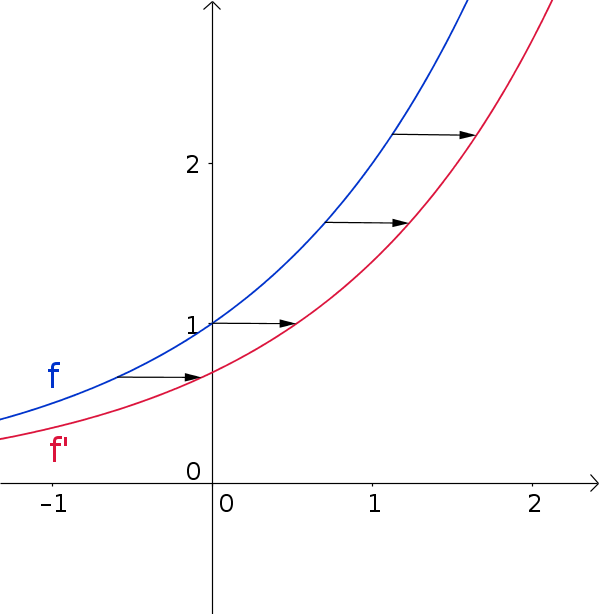Math Natural exponential function Derivatives of exponential functions

# Derivatives of exponential functions

When computing the derivative of general exponential functions one uses the natural logarithm.

!

### Remember

$f(x)=a^x$

$f'(x)=a^x\cdot\ln(a)$

### Example

$f(x)=2^x$
$f'(x)=2^x\cdot\ln(2)$When computing the derivative of an exponential function, it shifts along the x-axis.

### Derivation

Here the derivation of the phrase is described.

We are looking for the derivative of $f(x)=a^x$

1. #### Rewrite as exponential function

Since the ln-functionis the inverse of the exponential function, the following applies:

$x=e^{\ln(x)}$
$a^x=e^{\ln(a^x)}$

Now the logarithm law for powers is applied.
$a^x=e^{x\cdot\ln(a)}$

$f(x)=a^x=e^{x\cdot\ln(a)}$
2. #### Compute the derivative of the exponential function

$f(x)=e^{g(x)}$
$f'(x)=e^{g(x)}\cdot g'(x)$

$f(x)=e^{x\cdot\ln(a)}$
$f'(x)=e^{x\cdot\ln(a)}\cdot(x\cdot\ln(a))'$

$\ln(a)$is a constant factor (constant factor rule) and $(x)'=1$

$f'(x)=e^{x\cdot\ln(a)}\cdot\ln(a)$
3. #### Rewrite exponential function

Apply the method from the first step backwards:

$a^x=e^{x\cdot\ln(a)}$

$f'(x)=e^{x\cdot\ln(a)}\cdot\ln(a)$
$f'(x)=a^x\cdot\ln(a)$